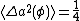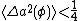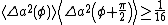# Squeezed quantum states

## Squeezed quantum states

Quantum states for which certain variables can be measured more accurately than is normally possible.

All matter and radiation fluctuate. Much random fluctuation derives from environmental influence, but even if all these influences are removed, there remains the intrinsic uncertainty prescribed by the laws of quantum physics. The position and momentum of a particle, or the electric and magnetic components of an electromagnetic field, are conjugate variables that cannot simultaneously possess definite values (Heisenberg uncertainty principle). It is possible, however, to have the position of a particle more and more accurately specified at the expense of increasing momentum uncertainty; the same applies to electromagnetic field amplitudes. This freedom underlies the phenomenon of squeezing or the possibility of having squeezed quantum states. With squeezed states, the inherent quantum fluctuation may be partly circumvented by focusing on the less noisy variable, thus permitting more precise measurement or information transfer than is otherwise possible. See Uncertainty principle

According to quantum electrodynamics, the vacuum is filled with a free electromagnetic field in its ground state that consists of fluctuating field components with significant noise energy. If &phgr; is a phase angle and a(&phgr; ) and a[&phgr; + (π/2)] are two quadrature components of the field (for example, the electric and magnetic field amplitudes), the vacuum mean-square field fluctuation is given by Eq. (1), independently of the phase

(1)angle.

Equation (1) is normalized to a photon; the corresponding equivalent noise temperature at optical frequencies is thousands of kelvins. Equation (1) also gives the general fluctuation of an arbitrary coherent state, which is the quantum state of ordinary lasers. Further environment-induced randomness is introduced in addition to Eq. (1) for other conventional light sources, including light-emitting diodes. See Coherence, Laser, Quantum electrodynamics

In a squeezed state, the quadrature fluctuation is reduced below Eq. (1) for some &phgr;, as given in

(2)Eq. (2). At that point, squeezing, that is, reduction of field fluctuation below the coherent state level, occurs. The fluctuation of the conjugate quadrature is correspondingly increased to preserve the uncertainty relation, Eq. (3). In a two-photon (3)coherent state, or squeezed state in the narrow sense, Eq. (3) is satisfied with equality. As seen in the illustration, the designation “squeezed state” is partly derived from the fact that the noise circle of Eq. (1) is squeezed to an ellipse when Eq. (3) is satisfied with equality.

Squeezed light can be generated by a variety of processes, especially nonlinear optical processes. The first successful experimental demonstration of squeezing, in 1985, involved a four-wave mixing process in an atomic beam of sodium atoms.

Squeezing was first studied in connection with optical communication, although it is evident that reduced quantum fluctuation might find applications in precision measurements. See Nonlinear optics

McGraw-Hill Concise Encyclopedia of Physics. © 2002 by The McGraw-Hill Companies, Inc.
Site: Follow: Share:
Open / Close